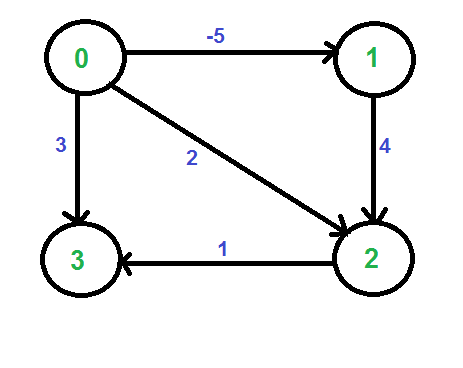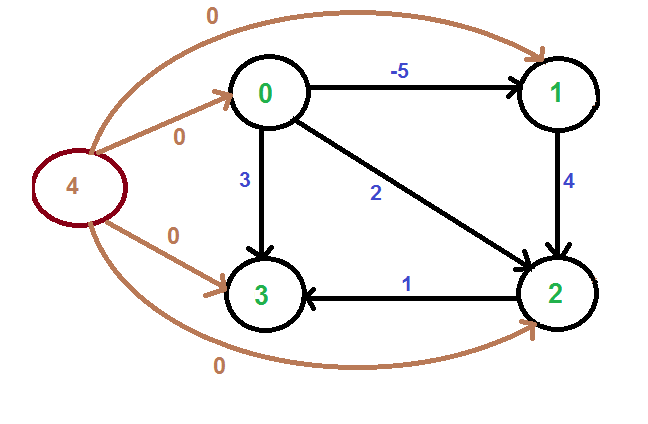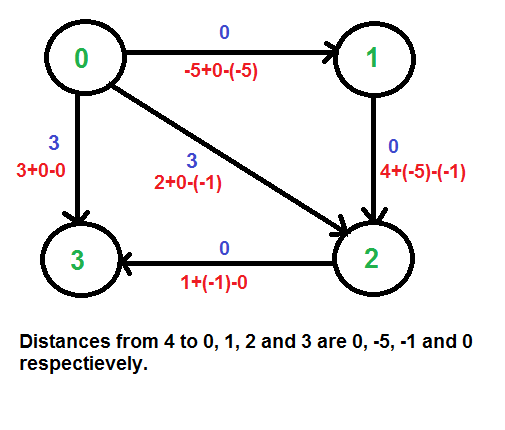# Johnson’s algorithm for All-pairs shortest paths | Implementation

Given a weighted Directed Graph where the weights may be negative, find the shortest path between every pair of vertices in the Graph using Johnson’s Algorithm.

The detailed explanation of Johnson’s algorithm has already been discussed in the previous post.

This post focusses on the implementation of Johnson’s Algorithm.

## Recommended: Please try your approach on {IDE} first, before moving on to the solution.

Algorithm:

1. Let the given graph be G. Add a new vertex s to the graph, add edges from new vertex to all vertices of G. Let the modified graph be G’.
2. Run Bellman-Ford algorithm on G’ with s as source. Let the distances calculated by Bellman-Ford be h, h, .. h[V-1]. If we find a negative weight cycle, then return. Note that the negative weight cycle cannot be created by new vertex s as there is no edge to s. All edges are from s.
3. Reweight the edges of original graph. For each edge (u, v), assign the new weight as “original weight + h[u] – h[v]”.
4. Remove the added vertex s and run Dijkstra’s algorithm for every vertex.

Example:
Let us consider the following graph.We add a source s and add edges from s to all vertices of the original graph. In the following diagram s is 4.We calculate the shortest distances from 4 to all other vertices using Bellman-Ford algorithm. The shortest distances from 4 to 0, 1, 2 and 3 are 0, -5, -1 and 0 respectively, i.e., h[] = {0, -5, -1, 0}. Once we get these distances, we remove the source vertex 4 and reweight the edges using following formula. w(u, v) = w(u, v) + h[u] – h[v].Since all weights are positive now, we can run Dijkstra’s shortest path algorithm for every vertex as source.

Below is the implementation of the above approach

 `# Implementation of Johnson's algorithm in Python3 ` ` `  `# Import function to initialize the dictionary ` `from` `collections ``import` `defaultdict ` `MAX_INT ``=` `float``(``'Inf'``) ` ` `  `# Returns the vertex with minimum  ` `# distance from the source ` `def` `minDistance(dist, visited): ` ` `  `    ``(minimum, minVertex) ``=` `(MAX_INT, ``0``) ` `    ``for` `vertex ``in` `range``(``len``(dist)): ` `        ``if` `minimum > dist[vertex] ``and` `visited[vertex] ``=``=` `False``: ` `            ``(minimum, minVertex) ``=` `(dist[vertex], vertex) ` ` `  `    ``return` `minVertex ` ` `  ` `  `# Dijkstra Algorithm for Modified  ` `# Graph (removing negative weights) ` `def` `Dijkstra(graph, modifiedGraph, src): ` ` `  `    ``# Number of vertices in the graph ` `    ``num_vertices ``=` `len``(graph) ` ` `  `    ``# Dictionary to check if given vertex is  ` `    ``# already included in the shortest path tree ` `    ``sptSet ``=` `defaultdict(``lambda` `: ``False``) ` ` `  `    ``# Shortest distance of all vertices from the source ` `    ``dist ``=` `[MAX_INT] ``*` `num_vertices ` ` `  `    ``dist[src] ``=` `0` ` `  `    ``for` `count ``in` `range``(num_vertices): ` ` `  `        ``# The current vertex which is at min Distance  ` `        ``# from the source and not yet included in the  ` `        ``# shortest path tree ` `        ``curVertex ``=` `minDistance(dist, sptSet) ` `        ``sptSet[curVertex] ``=` `True` ` `  `        ``for` `vertex ``in` `range``(num_vertices): ` `            ``if` `((sptSet[vertex] ``=``=` `False``) ``and` `                ``(dist[vertex] > (dist[curVertex] ``+`  `                ``modifiedGraph[curVertex][vertex])) ``and` `                ``(graph[curVertex][vertex] !``=` `0``)): ` `                 `  `                ``dist[vertex] ``=` `(dist[curVertex] ``+` `                                ``modifiedGraph[curVertex][vertex]); ` ` `  `    ``# Print the Shortest distance from the source ` `    ``for` `vertex ``in` `range``(num_vertices): ` `        ``print` `(``'Vertex '` `+` `str``(vertex) ``+` `': '` `+` `str``(dist[vertex])) ` ` `  `# Function to calculate shortest distances from source ` `# to all other vertices using Bellman-Ford algorithm ` `def` `BellmanFord(edges, graph, num_vertices): ` ` `  `    ``# Add a source s and calculate its min ` `    ``# distance from every other node ` `    ``dist ``=` `[MAX_INT] ``*` `(num_vertices ``+` `1``) ` `    ``dist[num_vertices] ``=` `0` ` `  `    ``for` `i ``in` `range``(num_vertices): ` `        ``edges.append([num_vertices, i, ``0``]) ` ` `  `    ``for` `i ``in` `range``(num_vertices): ` `        ``for` `(src, des, weight) ``in` `edges: ` `            ``if``((dist[src] !``=` `MAX_INT) ``and`  `                    ``(dist[src] ``+` `weight < dist[des])): ` `                ``dist[des] ``=` `dist[src] ``+` `weight ` ` `  `    ``# Don't send the value for the source added ` `    ``return` `dist[``0``:num_vertices] ` ` `  `# Function to implement Johnson Algorithm ` `def` `JohnsonAlgorithm(graph): ` ` `  `    ``edges ``=` `[] ` ` `  `    ``# Create a list of edges for Bellman-Ford Algorithm ` `    ``for` `i ``in` `range``(``len``(graph)): ` `        ``for` `j ``in` `range``(``len``(graph[i])): ` ` `  `            ``if` `graph[i][j] !``=` `0``: ` `                ``edges.append([i, j, graph[i][j]]) ` ` `  `    ``# Weights used to modify the original weights ` `    ``modifyWeights ``=` `BellmanFord(edges, graph, ``len``(graph)) ` ` `  `    ``modifiedGraph ``=` `[[``0` `for` `x ``in` `range``(``len``(graph))] ``for` `y ``in` `                    ``range``(``len``(graph))] ` ` `  `    ``# Modify the weights to get rid of negative weights ` `    ``for` `i ``in` `range``(``len``(graph)): ` `        ``for` `j ``in` `range``(``len``(graph[i])): ` ` `  `            ``if` `graph[i][j] !``=` `0``: ` `                ``modifiedGraph[i][j] ``=` `(graph[i][j] ``+`  `                        ``modifyWeights[i] ``-` `modifyWeights[j]); ` ` `  `    ``print` `(``'Modified Graph: '` `+` `str``(modifiedGraph)) ` ` `  `    ``# Run Dijkstra for every vertex as source one by one ` `    ``for` `src ``in` `range``(``len``(graph)): ` `        ``print` `(``'\nShortest Distance with vertex '` `+` `                        ``str``(src) ``+` `' as the source:\n'``) ` `        ``Dijkstra(graph, modifiedGraph, src) ` ` `  `# Driver Code ` `graph ``=` `[[``0``, ``-``5``, ``2``, ``3``],  ` `         ``[``0``, ``0``, ``4``, ``0``],  ` `         ``[``0``, ``0``, ``0``, ``1``],  ` `         ``[``0``, ``0``, ``0``, ``0``]] ` ` `  `JohnsonAlgorithm(graph) `

Output:

```Modified Graph: [[0, 0, 3, 3], [0, 0, 0, 0], [0, 0, 0, 0], [0, 0, 0, 0]]

Shortest Distance with vertex 0 as the source:

Vertex 0: 0
Vertex 1: 0
Vertex 2: 0
Vertex 3: 0

Shortest Distance with vertex 1 as the source:

Vertex 0: inf
Vertex 1: 0
Vertex 2: 0
Vertex 3: 0

Shortest Distance with vertex 2 as the source:

Vertex 0: inf
Vertex 1: inf
Vertex 2: 0
Vertex 3: 0

Shortest Distance with vertex 3 as the source:

Vertex 0: inf
Vertex 1: inf
Vertex 2: inf
Vertex 3: 0
```

Time Complexity: The time complexity of the above algorithm isas Dijkstra’s Algorithm takesfor adjacency matrix. Note that the above algorithm can be made more efficient by using adjacency list instead of the adjacency matrix to represent the Graph.

Attention reader! Don’t stop learning now. Get hold of all the important DSA concepts with the DSA Self Paced Course at a student-friendly price and become industry ready.

My Personal Notes arrow_drop_upCheck out this Author's contributed articles.

If you like GeeksforGeeks and would like to contribute, you can also write an article using contribute.geeksforgeeks.org or mail your article to contribute@geeksforgeeks.org. See your article appearing on the GeeksforGeeks main page and help other Geeks.

Please Improve this article if you find anything incorrect by clicking on the "Improve Article" button below.